# ECL Tips – The Seven Faces (Forms) of LOOP FUNCTION

The Enterprise Con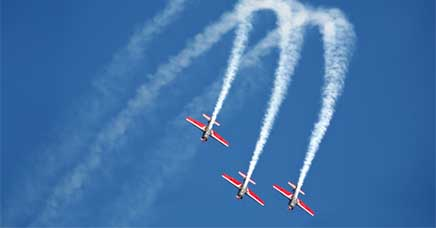trol Language (ECL) LOOP function has always been a powerful, yet tough function to understand and use. For this reason, changes were made to the HPCC Systems ECL Language Reference manual. The documentation updates make it easier to understand and effectively use the ECL LOOP function.

Bob Foreman, a technical trainer at LexisNexis® Risk Solutions, highlighted these changes during the HPCC Systems Tech Talk 20, as part of his monthly “ECL Tips.” Bob is a frequent contributor to our Tech Talks, and provides valuable information on programming with Enterprise Control Language (ECL).

In this blog, we:

• Discuss the ECL LOOP Function
• Examine the changes to the ECL LOOP function documentation.
• Provide examples of how to use the various forms of the ECL LOOP function.

ECL LOOP Function is Not a Typical Loop
The Enterprise Control Language (ECL) LOOP function does not operate as a typical LOOP. ECL LOOP functionality can best be described as a form of recursion. Recursion is used when a problem is too complex to write as iterative code. This is normally due to a large amount of data to be processed. In recursion a function, algorithm, or sequence of instructions loops back to the beginning of itself until it detects that some condition has been satisfied.

Updated Documentation for the ECL LOOP Function
In the updated ECL LOOP function documentation, the LOOP function syntax diagram has been simplified to a single line:

`` LOOP( dataset,  [ loopcount | loopfilter | loopcondition ] , loopbody [, UNORDERED | ORDERED( bool ) ] [, STABLE | UNSTABLE ] [, PARALLEL [ numthreads ) ] ] [, ALGORITHM( name ) ][, FEW] )``

The current documentation clarifies the use of the ECL LOOP function and all associated parameters.

When applying the updated ECL LOOP forms:

• Loopbody must be used whenever the LOOP function is used, but the loopcount, loopfilter, and loopcondition parameters are all optional.
• At least one of the three middle parameters (loopcount, loopfilter, loopcondition) must be present.
• Combinations of these parameters can be used. Considering all possible combinations of these three parameters, there are now seven forms the ECL LOOP function can take.

New ECL LOOP Forms
Let’s look at the updated ECL LOOP forms and examples of how they can be applied. Please note that the LOOP forms are represented differently in the ECL Language Reference Manual. For illustration purposes, these forms have been extracted and represented as separate running examples.

You are encouraged to download these examples, modify the parameters, and see how the output changes.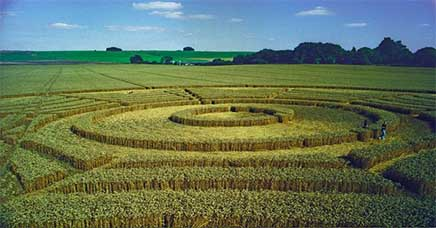Form 1
LOOP(ds, loopcount, loopbody)

Form 1 iterates loopcount times. This is basically a “for loop” construct.

Example 1

``namesRec := RECORD  STRING20  lname; STRING10  fname; UNSIGNED2 age := 25; UNSIGNED2 ctr := 0;END;namesTable := DATASET([{'Flintstone','Fred',35},                        {'Flintstone','Wilma',43},                         {'Jetson','Georgie',10},                        {'Mr. T','Z-man'}], namesRec);BodyFunc(DATASET(namesRec) ds, UNSIGNED4 c):= PROJECT(ds,          TRANSFORM(namesRec,                    SELF.age := LEFT.age*c;                    SELF.ctr := COUNTER*c ;                    SELF := LEFT));Form1 := LOOP(namesTable, 2,           //iterate 2 times               ROWS(LEFT) & BodyFunc(ROWS(LEFT),               COUNTER));//16 rows: OUTPUT(Form1,NAMED('Form1_example'));  ``

Form 1 combines a loopcount with a loopbody. In example 1, the PROJECT function calls its inline TRANSFORM once for each record in the passed DATASET parameter. The first LOOP iteration passes the starting dataset (namesTable) to the PROJECT, then the second LOOP iteration passes the result record set from the first iteration. The loopbody takes an expression in the “ROWS(LEFT)” with “BodyFunc(ROWS(LEFT), “and also passes the COUNTER.  The initial dataset here is four rows, but each loop processes eight rows at a time. The first iteration produces eight rows (the first four are the input records and the second four are the result from the BodyFunc), and the second iteration starts with those eight rows from the first iteration and does the same process again. The aggregate results are the 16 records returned from the second iteration.

An interesting note is that there is a COUNTER parameter in the Form1 function, but the PROJECT function also uses a COUNTER. The COUNTER in the TRANSFORM function counts the number of records processed by the current PROJECT iteration, but the COUNTER in the LOOP function determines the number of iterations the LOOP has completed.

In the first iteration, the original four records have a COUNTER that is initialized to zero, per the definition “UNSIGNED2 ctr := 0; .” The BodyFunc function projects through the first four records and gives us a one, two, three, four count for records five thru eight. The “ctr” calculation is defined as “SELF.ctr := COUNTER*c” in the TRANSFORM function. The result is COUNTER*c (record number times iteration), or 1*1, 2*1, 3*1, 4*1. The “age” calculation is defined as “SELF.age := LEFT.age*c” in the TRANSFORM function. The ages are multiplied by 1, since “c” (iteration) is equal to 1.

The second iteration, which processes records nine thru sixteen, processes through the first eight records, but the COUNTER in the TRANSFORM function starts at one. So, for records nine through twelve, it takes COUNTER one, two, three, and four, and multiplies by two (1*2 is 2, 2*2 is four, 3*2 is six, 4*2 is eight). The iteration for records thirteen thru sixteen takes COUNTER five, six, seven, and eight and multiplies by two (5*2 is 10, 6*2 is 12, etc.). The ages are multiplied by 2 since “c” (iteration) = 2.The output table for example 1 is found below:

Output Table 1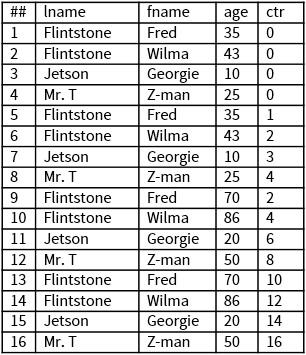Form 2
LOOP(ds, loopfilter, loopbody

Form 2 continues processing while the loopfilter expression is TRUE for any records in ROWS(LEFT). This is basically a “while loop” construct. The loopfilter expression is evaluated on the entire set of ROWS(LEFT) records prior to each iteration.

Example 2

``namesRec := RECORD STRING20 lname; STRING10 fname; UNSIGNED2 age := 25; UNSIGNED2 ctr := 0;END;namesTable :=DATASET([{'Flintstone','Fred',35},                   {'Flintstone','Wilma',43},                   {'Jetson','Georgie',10},                    {'Mr. T','Z-man'}], namesRec);Form2 := LOOP(namesTable, LEFT.age < 100,//process only recs where expression is TRUE              PROJECT(ROWS(LEFT),                  TRANSFORM(namesRec,                      SELF.age := LEFT.age*2;                      SELF     := LEFT)));OUTPUT(Form2,NAMED('Form2_example')); ``

Form 2 combines a loopfilter with a loopbody. In example 2, the PROJECT function calls its inline TRANSFORM once for each record in the passed DATASET parameter. The first LOOP iteration passes the starting dataset (namesTable) to the PROJECT, where the TRANSFORM function defines, “SELF.age := LEFT.age*2.” Each subsequent iteration passes the result record set from the prior iteration, and the Form2 function continues processing as long as the loopfilter expression, “LEFT.age < 100,” is TRUE for any records in “ROWS(LEFT).” The loopfilter selectively only processes records that match the filter.

Let’s look at the first record to understand the processing for this example.  Fred Flintstone has an initial “age” of 35. That “age” is multiplied by 2 and becomes 70, per the TRANSFORM function definition “SELF.age := LEFT.age*2.” Since 70 is less than 100, as defined by the loopfilter definition, “LEFT.age < 100,” there is a second iteration, where “SELF.age “= 70*2= 140. There are no further iterations for that record after the 2nd iteration, because the “LEFT.age” reached 140, and the loopfilter expression, “LEFT.age < 100,” is no longer TRUE. Note that there are no definitions for the “ctr” expression.

Now let’s look at the record for Georgie Jetson. His initial “age” is 10. In the first iteration, that “age” is multiplied by 2 and becomes 20. According to the loopfilter expression, “LEFT.age <100,” which meets the loopfilter condition.  In the second iteration, 20 is multiplied by 2 and becomes 40. The “LEFT.age” is still less than 100, so we go to a third iteration, where 40*2 = 80. We still haven’t reached the loopfilter limit of 100, so a fourth iteration takes place, where 80*2=160. No further processing will occur after the 4th iteration, since the loopfilter condition is no longer TRUE. The output table for example 2 is shown below:

Output Table 2Form 3:
LOOP(ds, loopcondition, loopbody

Form 3 continues processing while the loopcondition expression is TRUE. This is basically a “while loop” construct. The loopcondition expression is evaluated on the entire set of “ROWS(LEFT)” records prior to each iteration.

Example 3

``namesRec := RECORD  STRING20 lname;namesTable :=  DATASET([{'Flintstone','Fred',35},          {'Flintstone','Wilma',43},          {'Jetson','Georgie',10},          {'Mr. T','Z-man'}], namesRec);Form3 := LOOP(namesTable,         SUM(ROWS(LEFT), age)<1000*COUNTER,         PROJECT(ROWS(LEFT),            TRANSFORM(namesRec,                     SELF.age := LEFT.age*2;SELF   := LEFT)));OUTPUT(Form3,NAMED('Form3_example')); ``

Form 3 combines a loopcondition with a loopbody. In example 3, the PROJECT function calls its inline TRANSFORM once for each record in the passed DATASET parameter. The first LOOP iteration passes the starting dataset (namesTable) to the PROJECT, where the TRANSFORM function defines “SELF.age := LEFT.age*2.” The loopcondition “SUM(ROWS(LEFT), age) < 1000 * COUNTER “ is evaluated on the entire set of “ROWS(LEFT)” records prior to each iteration, and continues processing until the loopcondition is no longer TRUE. Processing continues only if the loopcondition is TRUE for all records in the record set, unlike the loopfilter, which selectively processes records that match the filter condition.

Let’s consider the evaluation for Fred Flintstone. His starting “age” is 35. After the first iteration, the calculation for the “age” expression is 35*2=70, as defined by “SELF.age := LEFT.age*2.” The “SUM(ROWS(LEFT)” = 70, and “1000*COUNTER” =1000, where the COUNTER is the number of iterations the LOOP has completed. For iteration 2, Fred Flintstone’s “age” is 70*2= 140, which is the “LEFT.age” (“age” for the prior iteration) times 2. The “SUM(ROWS(LEFT)” calculation is the “ROWS(LEFT)” value for the prior iteration + the “age” for the current iteration, so 70 + 140 = 210. For “1000*COUNTER,” the calculation is 1000 *2= 2000. The iterations continue until the “age” reaches 2240. The loopcondition “SUM(ROWS(LEFT), age) < 1000 * COUNTER “ is no longer TRUE after iteration 6, therefore all processing stops. Note that the iterations stop as soon as any record in the record set fails to meet the loopcondition. In this case, processing for all records stop after the 6th LOOP.  The output table for example 3 is shown below.

Output Table 3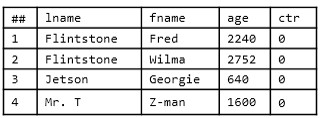Form 4:
LOOP(ds, loopcount, loopfilter, loopbody).

Form 4 processes loopcount times, with the loopfilter expression defining when each record continues to process through the loopbody expression. This is basically a “for loop” construct with a filter specifying which records are processed each iteration.

Example 4

``namesRec := RECORD  STRING20 lname;  STRING10 fname;  UNSIGNED2 age := 25;  UNSIGNED2 ctr := 0; END;namesTable := DATASET([{'Flintstone','Fred',35},                       {'Flintstone','Wilma',43},                       {'Jetson','Georgie',10},                       {'Mr. T','Z-man'}], namesRec);BodyFunc(DATASET(namesRec) ds, UNSIGNED4 c) :=   PROJECT(ds,      TRANSFORM(namesRec,          SELF.age := LEFT.age*c;          SELF.ctr := COUNTER*c ;          SELF := LEFT)); Form4 := LOOP(namesTable,               10,               LEFT.age < 100, //process only TRUE recs               BodyFunc(ROWS(LEFT), COUNTER));OUTPUT(Form4,NAMED('Form4_example'));  ``

Form 4 combines a hard-coded loopcount and loopfilter with a loopbody. In example 4, the PROJECT function calls its inline TRANSFORM once for each record in the passed DATASET parameter. The first LOOP iteration passes the starting dataset (namesTable) to the PROJECT, where the TRANSFORM function defines, “SELF.age := LEFT.age*c.” (“c” is the current PROJECT iteration).  There is a hard-coded loopcount in Form4 equal to 10, along with a loopfilter condition, “LEFT.age < 100, //process only TRUE recs.” This loopfilter specifies which records are processed during each iteration. Each iteration passes the result record set from the prior iteration, and the Form4 function continues processing until the loopfilter expression is either no longer TRUE, or, according to the loopcount expression, reaches 10 iterations.

Let’s consider the evaluation for Fred Flintstone. His starting “age” is 35. So, after the first iteration, the calculation for the “age” is 35*1=35, as defined by “SELF.age := LEFT.age*c.” The “ctr”=1*1=1, per the definition “SELF.ctr := COUNTER*c,” and the “LEFT.age” = 35, which is less than 100. For iteration 2, the “age”=35*2, which equals 70. The “ctr”=1*2=2, and the “LEFT.age”=35, which is less than 100, so we move to a third iteration. In the 3rd iteration, the “SELF.age”=70*3=210, the “ctr”=1*3=3. If we try to move to a 4th iteration, the LOOP is broken because the “LEFT.age”=210, which makes the loopfilter FALSE. So, the LOOP stops for that record.

The result record sets for Fred Flintstone, Wilma Flintstone, and Mr. T no longer meet the loopfilter definition “LEFT.age<100” after the 3rd LOOP, so calculations stop for those three records. Georgie Jetson still has a “LEFT.age <100” that meets the TRUE condition after the 3rd iteration, so calculations continue for a 4th iteration, where that one record is processed. This means that the COUNTER goes to 1, so the “SELF.ctr:=COUNTER*c” calculation is 1*4=4, and “SELF.age” = 60*4, which equals 240. If we try to go to a 5th iteration, “LEFT.age”=240, which means that the loopfilter is FALSE. So, processing stops for Georgie Jetson stop after the 4th LOOP. The output table for example 4 is shown below.

Output Table 4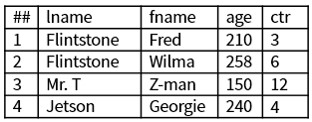Form 5:
LOOP(ds, loopcount, loopcondition, loopbody

Form 5 processes loopcount times, with the loopcondition expression defining the set of records that continue to process through the loopbody expression. This is basically a “for loop” construct with a filter specifying the record set processed for each iteration.

Example 5

``namesRec := RECORD  STRING20 lname;  STRING10 fname;  UNSIGNED2 age := 25;  UNSIGNED2 ctr := 0;END;namesTable := DATASET([{'Flintstone','Fred',35},                       {'Flintstone','Wilma',43},                       {'Jetson','Georgie',10},                       {'Mr. T','Z-man'}], namesRec);Form5 := LOOP(namesTable,               10,             //iterate 10 times    LEFT.age * COUNTER <= 200, //process only TRUE recs          PROJECT(ROWS(LEFT),            TRANSFORM(namesRec,                      SELF.age := LEFT.age*2,                      SELF.ctr := COUNTER,                      SELF := LEFT)));OUTPUT(Form5,NAMED('Form5_example')) ``

Form 5 combines a loopcount  and loopcondition with a loopbody. In example 5, there is an inline loopbody that uses the PROJECT function. The PROJECT function calls its inline TRANSFORM once for each record in the passed DATASET parameter. The first LOOP iteration passes the starting dataset (namesTable) to the PROJECT, where the TRANSFORM function defines “SELF.age := LEFT.age*2.” The loopcondition expression, “LEFT.age * COUNTER <= 200, //process only TRUE recs,” specifies which records are processed during each iteration, and continues processing until the loopcondition is no longer TRUE. There is a hard-coded loopcount of 10, but if the loopcondition, “LEFT.age * COUNTER <= 200,” fails before 10 iterations, the LOOP will break early.

Let’s look at calculations for Fred Flintstone. The starting “age” for this calculation is 35*2=70, as defined by the expression “SELF.age := LEFT.age*2.” After the first LOOP the “age” is 70*2 = 140. The “ctr” =1 for record 1, since “SELF.ctr := COUNTER,”  and the COUNTER is the number of records processed by the current iteration. If we go to the loopcondition, “LEFT.age*COUNTER” = 140*1=140. Note that the COUNTER in the loopcondition expression is the number of iterations the LOOP has completed. In this case, the loopcondition is TRUE, since 140 is less than 200. If we try to go to a 2nd iteration, the loopcondition is FALSE (LEFT.age * COUNTER=140*2= 280, and 280 is greater than 200). Iterations for Wilma Flintstone and Mr. T also stop after the 1st LOOP because of their failure to meet the loopcondition

If we try to move on to a 3rd iteration for Georgie Jetson, the loopcondition, “LEFT.age*COUNTER”= 80*3=240. The loopcondition is no longer TRUE, therefore, calculations for Georgie Jetson stop after the 3rd iteration. The output table for example 5 is shown below.

Output Table 5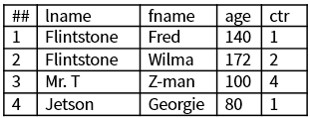Form 6:

LOOP(ds, loopfilter, loopcondition, loopbody)

Form 6 continues processing while the loopcondition expression is TRUE. Records where the loopfilter expression is TRUE continue processing. This is basically a “while loop” construct with individual record processing continuation logic.

Example 6

``namesRec := RECORD  STRING20 lname;  STRING10 fname;  UNSIGNED2 age := 25;  UNSIGNED2 ctr := 0;END;namesTable := DATASET([{'Flintstone','Fred',35},                       {'Flintstone','Wilma',43},                       {'Jetson','Georgie',10},                       {'Mr. T','Z-man'}], namesRec);BodyFunc(DATASET(namesRec) ds, UNSIGNED4 c) :=   PROJECT(ds,        TRANSFORM(namesRec,          SELF.age := LEFT.age*c;          SELF.ctr := COUNTER*c ;          SELF := LEFT));Form6 := LOOP(namesTable,               LEFT.age < 100,               EXISTS(ROWS(LEFT)) AND               SUM(ROWS(LEFT), age) < 1000,               BodyFunc(ROWS(LEFT), COUNTER));OUTPUT(Form6,NAMED('Form6_example')); ``

Form 6 combines a loopfilter and loopcondition with a loopbody.  In example 6, the PROJECT function calls its inline TRANSFORM once for each record in the passed DATASET parameter. The first LOOP iteration passes the starting dataset (namesTable) to the PROJECT, where the TRANSFORM function defines “SELF.age := LEFT.age*c,” and “SELF.ctr := COUNTER*c”. The 2nd LOOP iteration passes the result record set from the first iteration. The loopbody takes an expression in the “ROWS(LEFT)” with “BodyFunc(ROWS(LEFT),” and also passes the COUNTER. The LOOP iterations continue, with the loopfilter selectively processing records that match the “LEFT.age < 100” filter, and the loopcondition, “EXISTS(ROWS(LEFT)) AND  SUM(ROWS(LEFT), age) < 1000”.

Example 6 also demonstrates the two possible scopes of the COUNTER keyword within a LOOP.

• The COUNTER in the LOOP function (passed to BodyFunc) is the number of iterations the LOOP has done.
• The COUNTER in the TRANSFORM for the PROJECT in the BodyFunc counts the number of records processed by the current PROJECT iteration.

Let’s consider the evaluation for Fred Flintstone. His starting “age” is 35. So, after the first iteration, the calculation for the “age” is 35*1=35, as defined by “SELF.age := LEFT.age*c.” The “SUM(ROWS(LEFT)” = 35, which is less than 1000. The loopfilter and loopcondition expressions are TRUE, so we go to a 2nd iteration. In iteration 2, the “age” is 70 (which is the “age” for the prior iteration) times 2, per definition “SELF.age := LEFT.age*c.” The “LEFT.age”=35, which is less than 100, and the “SUM(ROWS(LEFT)” calculation is the “ROWS(LEFT)” from the prior iteration + the “age” for the current iteration, so 35 + 70 = 105, which is less than 1000. Both the loopfilter and loopcondition expressions are TRUE so we move to a third iteration. In the 3rd iteration, the “SELF.age”=70*3=210, the “SUM(ROWS(LEFT)”=70+210=280, and the “LEFT.age = 70,” which is less than 100. If we try move to a 4th iteration, the LOOP is broken because the “LEFT.age=210,” which makes the loopfilter FALSE. So, the iterations stop after the 3rd LOOP for that record.

The result record sets for Fred Flintstone, Wilma Flintstone, and Mr. T no longer meet the loopfilter definition “LEFT.age<100” after the 3rd iteration, so calculations stop. Georgie Jetson still has a “LEFT.age ” that meets the TRUE condition after the 3rd iteration, so calculations continue for a 4th iteration, with that one record being processed. This means that the COUNTER goes to 1, so the “SELF.ctr := COUNTER*c” calculation is 1*4=4, and “SELF.age” = 60*4=240. The loopfilter is no longer TRUE after the 4th iteration, so, calculations for Georgie Jetson stop. The output table for example 6 is shown below.

Output Table 6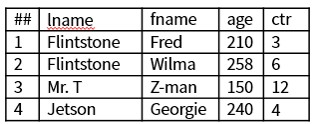Form 7:
LOOP(ds, loopcount, loopfilter, loopcondition, loopbody)

Form 7 continues processing while the loopcondition expression is TRUE. Records where the loopfilter expression is TRUE continue processing. This is basically a “while loop” construct with individual record processing continuation logic.

Example 7

``namesRec := RECORD  STRING20 lname;  STRING10 fname;  UNSIGNED2 age := 25;  UNSIGNED2 ctr := 0;END;namesTable := DATASET([{'Flintstone','Fred',35},                       {'Flintstone','Wilma',43},                       {'Jetson','Georgie',10},                       {'Mr. T','Z-man'}], namesRec);BodyFunc(DATASET(namesRec) ds, UNSIGNED4 c) :=   PROJECT(ds,           TRANSFORM(namesRec,         SELF.age := LEFT.age*c;         SELF.ctr := COUNTER*c ;         SELF := LEFT));Form7 := LOOP(namesTable,               10,               LEFT.age < 100,               EXISTS(ROWS(LEFT)) AND               SUM(ROWS(LEFT), age) < 1000,               BodyFunc(ROWS(LEFT), COUNTER));OUTPUT(Form7,NAMED('Form7_example')); ``

Form 7 combines loopcount, loopfilter, loopcondition, and loopbody. In example 7, the PROJECT function calls its inline TRANSFORM once for each record in the passed DATASET parameter. The first LOOP iteration passes the starting dataset (namesTable) to the PROJECT, where the TRANSFORM function defines “SELF.age := LEFT.age*c,” and “SELF.ctr := COUNTER*c”. The 2nd LOOP iteration passes the result record set from the first iteration. The loopbody takes an expression in the “ROWS(LEFT) with BodyFunc(ROWS(LEFT),” and also passes the COUNTER. The LOOP iterations continue, with the loopfilter selectively processing records that match the “LEFT.age < 100” filter, and the loopcondition expression, “EXISTS(ROWS(LEFT)) AND  SUM(ROWS(LEFT), age) < 1000”. The loopcount has a hard-count of 10. However, if the loopfilter or loopcondition is FALSE before LOOP number 10, then the iterations immediately stop.

Let’s look at the calculations for Fred Flintstone. His starting “age” is 35, so after the first iteration, the calculation for the “age” expression is 35*1=35, as defined by “SELF.age := LEFT.age*c”. The “SUM(ROWS(LEFT)” = 35, and “SELF.ctr := COUNTER*c”, which is 1*1 = 1. For iteration 2, the “age”=35*2=70 The “SUM(ROWS(LEFT), age” calculation is the  “ROWS(LEFT)” for the prior iteration + the “age” for the current iteration, so 70 + 140 = 210. The calculation “1000 *COUNTER”= 2000 for the second iteration. The iterations continue until the “age” reaches 2240. The loopcondition, “SUM(ROWS(LEFT), age” < 1000 * COUNTER, “ is FALSE after LOOP 3, so all processing stops.

The result record sets for Fred Flintstone, Wilma Flintstone, and Mr. T no longer meet the loopfilter condition, “LEFT.age<100,” after the 3rd iteration, so iterations stop for those three records. Georgie Jetson still has a result record set that meets the loopfilter and loopcondition, so calculations continue for a 4th iteration, where that one record is processed. The COUNTER goes to 1, so the “ctr” calculation is 1*4=4, and the “age” =60*4=240. The “SUM(ROWS(LEFT), age”=350, so the loopcondition is TRUE. However, the loopfilter condition is FALSE after the 4th iteration, so the LOOP stops. The output table for example 7 is shown below:

Output Table 7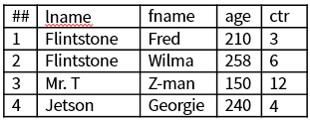Summary
The ECL LOOP function is a wonderful tool when iterations are necessary for large amounts of data. The updated documentation in the ECL Language Reference Manual makes it much easier to understand and choose the correct LOOP form for your needs.

More information about the HPCC Systems Enterprise Control Language (ECL) can be found in the ECL Language Reference Manual. Please use the link below to access the document.

Since 2011, Bob Foreman has worked with the HPCC Systems technology platform and the ECL programming language, and has been a technical trainer for over 25 years. He is the developer and designer of the HPCC Systems Online Training Courses, and is the Senior Instructor for all classroom and WebEx/Lync based training.

If you would like to watch Bob Foreman’s Tech Talk video on the ECL LOOP Function, please use the following link:

Acknowledgements
A special thank you goes to Bob Foreman and Richard Taylor for their guidance and valuable contributions to this blog post.